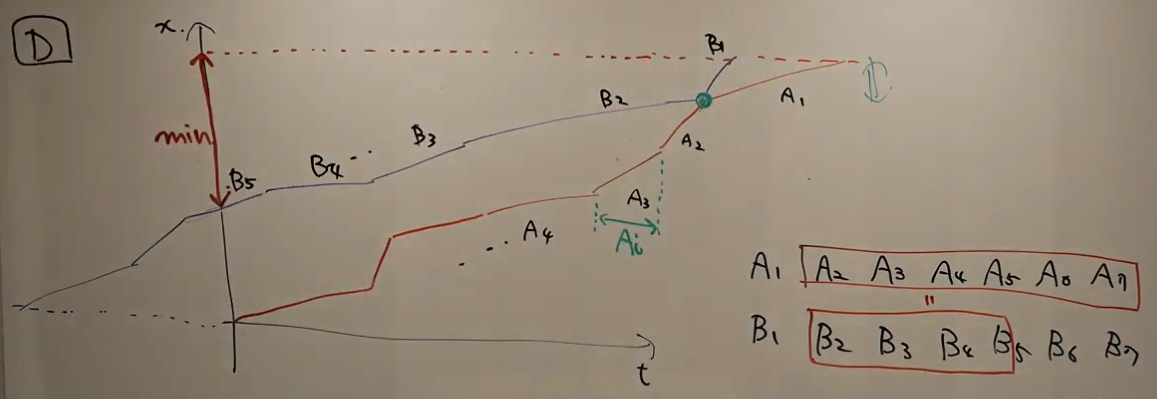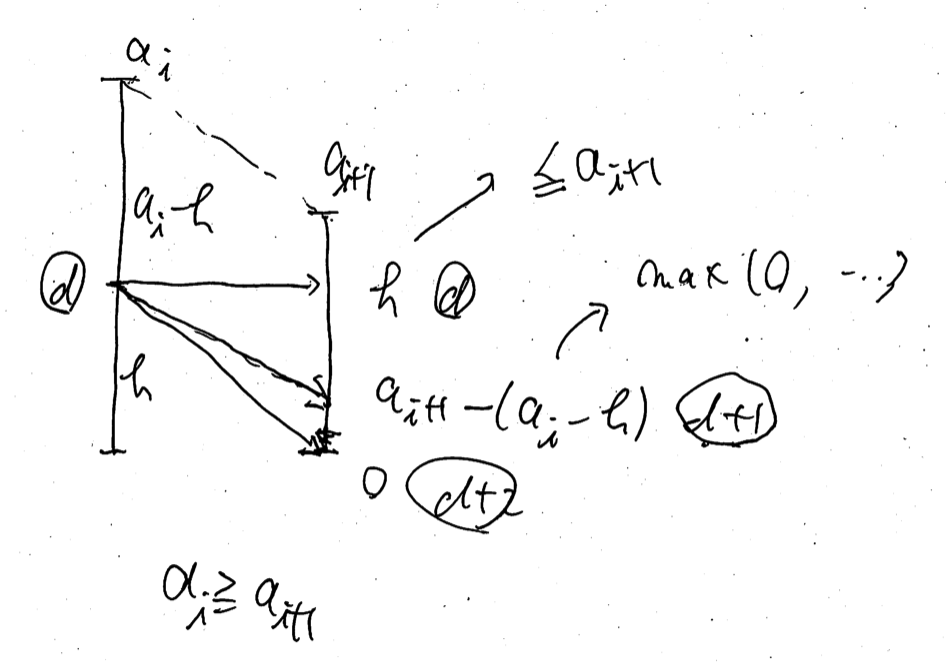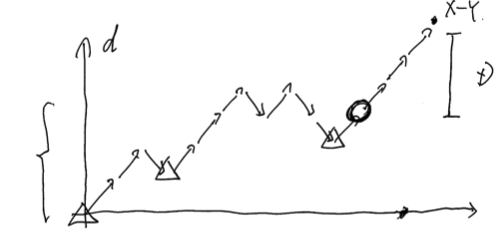# AtCoder Grand Contest 040

Updated:

AtCoder Grand Contest 040

# Solutions

## A - ><

### Solution

First, we initialize vector<ll> V(N, 0LL); as a candidate for good sequences. We divide this problems into two parts as follows.

• For all $i \in (N - 1)$ with $S[i] = “<”$, we change $a _ {i + 1}$ so that $a _ i < a _ {i + 1}$ in ascending order.
• For all $i \in (N - 1)$ with $S[i] = “>”$, we change $a _ {i}$ so that $a _ i > a _ {i + 1}$ in descending order.

This greedy algorithm works. We can solve this problem in $O(2N) = O(N)$-time.

## B - Two Contests

### Solution

We focus on $x = \mathop{\mathrm{argmin} } _ i R _ i$ and $y = \mathop{\mathrm{argmax} } _ i L _ i$. If $x = y$, or $x$ and $y$ are included in the same contest, in this contest the number of the satisfied people must be $\max(0, R _ x - L _ y)$. Thus the best strategy is that we choose $z = \mathop{\mathrm{argmax} } _ {i \in N \setminus \{ x, y \} } R _ i - L _ i$ and let the other contest consist of only one problem $z$. Let $ans _ 0$ be the answer by this case.

So we consider otherwise, that is, we consider, given $x \neq y$, the one contest $X$ includes $x$ and the other $Y$ includes $y$. Let’s see what is the best strategy here. Let $V = N \setminus \{ x, y \}$. and sort $I = \{ (L _ i, R _ i) \} _ {i \in V}$ in descending order of $R _ i$. We choose some ranges from $I$ to push into $Y$. What is the best strategy here?

Let’s focus on $r = \min _ {i \in B} R _ i$. To make the argument simple, we fix $r$. For $e = (L _ i , R _ i) \in I$, If $R _ i \geq r$, we should push $e$ into $B$ because if we push $e$ into $B$, we don’t lose anyone satisfied, but if we push $e$ into $A$, we may or may not lose those, which is not the best strategy. On the other hand, if $R _ i < r$, we must push $e$ into $A$; or otherwise it contradicts the definition of $r$. Therefore we conclude that our best strategy is that trying all $0 \leq k \leq N - 2$. We push $(L _ i, R _ i) \in I$ into $B$ if $i < k$ and into $A$ if $i \geq k$.

Let’s discuss the time complexity. Sorting $I = \{ (L _ i, R _ i) \} _ {i \in V}$ in descending order of $R _ i$ takes $O(N \log N)$-time. We can compute all $r _ k = \min _ {i < k} R _ i$ and $l _ k = \max _ {i \geq k} L _ i$ by partial min/max method in $O(N)$-time. The answer is $\max \left( ans _ 0, \max _ {0 \leq k \leq N - 2} \left( \max\left(0, R _ x - l _ k \right) + \max\left(0, r _ k - L _ y \right) \right) \right).$

## C - Neither AB nor BA

### Solution

We translate $s$ into $S$ as follows. $S[i] = \begin{cases} s[i] & i \text{ is even}, \\ B & i \text{ is odd and } s[i] = A, \\ A & i \text{ is odd and } s[i] = B, \\ C & i \text{ is odd and } s[i] = C. \end{cases}$

The map $s \mapsto S$ is a bijection. So we count the number of good $S$. In $S$, the forbidden pairs of characters are $AA$ and $BB$. Hereinafter, we use $A, B, C$ to denote the number of A, B, C in $S$, respectively.

The number of the manipulations must be $N / 2$. Thus we see that, if $A > N / 2$ or $B > N / 2$, we cannot reduce $S$ into the empty string. However, otherwise, we can reduce $S$ into the empty string. Why? Let’s consider the case where $A = B = N / 2$. Then, we can find a place where A and B are adjacent to each other. We eliminate it. Then the problem becomes smaller than the original problem. We can continue this manipulation $N / 2$ times. Other cases are almost the same. We regard each C as A or B virtually so that $A = B = N / 2$. Then the same strategy works.

Let $X$ be the number of $S$ that satisfies $A > N / 2$. The case $B > N / 2$ is the same. The answer is $3 ^ N - 2X$. Here, $X$ is computed as follows. $X = \sum _ {N / 2 + 1 \leq k \leq N} \begin{pmatrix} N \\ k \end{pmatrix} 2 ^ {N - k}.$

## D - Balance Beam

### Observation

Let’s see this problem from the viewpoint of two graphs. Let the $x$-axis denote the time and the $y$-axis denote the distance from the starting point. We draw two graphs and let $I = \{ (A _ i, B _ i) \} _ {i \in N}$ be ordered as the following picture (from the official YouTube live).Then, the problem will be translated as follows.

Problem D.1: Rearrange $I$ to minimize $(M, r) \in N \times [0, 1)$ so that there exists $k \in N$ so that $\sum _ {i = k} ^{N - 1} A _ i \leq \sum _ {i = k} ^ {M - 1} B _ i + r B _ M. \tag{D.1}$

Let $C _ i = \max(A _ i, B _ i)$. We will show that Problem D.1 is equivalent to the following problem.

Problem D.2: Rearrange $I$ to minimize $(M, r) \in N \times [0, 1)$ so that $\sum _ {i = 0} ^{N - 1} A _ i \leq \sum _ {i = 0} ^ {M - 1} C _ i + r B _ M. \tag{D.2}$

Let $(M, r) \in N \times [0, 1)$ satisfy the constraints of Problem D.1. There exists $k \in N$ satisfying (D.1). Then, (D.2) immediately follows by the definition of $C _ i$. Next, let $(M, r) \in N \times [0, 1)$ satisfy the constraints of Problem D.2. We can rearrange the order of $I$ so that there exists $1 \leq k \leq N$ so that $C _ i = \begin{cases} A _ i & i < k, \\ B _ i & i \geq k. \end{cases}$ Then, (D.2) becomes (D.1), which completes the proof.

### Solution

We can solve Problem D.2 to try all $B _ i$ for $b = B _ M$. Fix $b = B _ M$. Then, the best strategy to minimize $M$ is that we choose $C _ i$ in the descending order. We can do this greedy algorithm in $O(\log N)$-time for each $b$ by binary search if we prepare partial sums in $O(N)$-time. Then, we compute $r$ as follows. $r = \max \left(0, \frac{\sum _ {i = 0} ^ {N - 1} A _ i - \sum _ {i = 0} ^ {M - 1} C _ i}{B _ M} \right).$ In this case, the answer is $\frac{N - M - r}{N}.$ We compute the maximum of them.

We have to care one type of corner cases. If we have $\sum _ {i = 0}^ {N - 1} A _ i = \sum _ {i = 0}^ {N - 1} C _ i,$ the answer is 0 1. These cases are not necessarily corner cases if the implement is good, but be careful.

## E - Prefix Suffix Addition

We use $a[i]$ instead of $A[i]$. I should have made correct pictures. We use $0$-indexed, but, without loss of generality, we insert $a = 0$ and $a[N + 1] = 0$.

### Preliminary

We are given two operations. But first of all, we consider only “Operation 1”. How many times we need to meet the objective? Of course we can construct arbitrary $\{ a[i] \}$ by $N$ times. Just make $a[i]$ for each manipulation. But we may or may not reduce the number of it. Let’s consider the following sequence.

$0, 2, 1, 3, 4, 6, 5, 0.$

Here we point out “$2, 1$”. We need at least a pair of operations to make this since $2 > 1$. We can find two more points: $6 > 5 > 0$. So we have to use the operation at least three times.

On the other hand, three times is suffice to make this sequence. We can construct as follows. \begin{align} & 0, 2, \\ & 0, 0, 1, 3, 4, 6, \\ & 0, 0, 0, 0, 0, 0, 5. \end{align}

Generally speaking, we can show that, to obtain the sequence $\{ a[i] \}$, we need exactly $x$ operations, where $x$ is the number of $i$ with $a[i] > a[i + 1]$.

We can say the same thing for “Operation 2”. we need $y$ times, where $y$ is the count of “$<$”.

### Translate the problem into another

By the observation above, we see that all we have to do is solve the following problem.

Problem E.1: We will split each $a[i]$ into two nonnegative integers $b[i]$ and $c[i]$ with $a[i] = b[i] + c[i]$. Find the minimum count of $i$ with $b[i] > b[i + 1]$ plus $i$ with $c[i] < c[i + 1]$.

We regard an “$i$ with $b[i] > b[i + 1]$” and an “$i$ with $c[i] < c[i + 1]$” as a penalty. We regard the number of the accumulated penalties as a distance.

It is natural for us to convert Problem E.1 into DP. Obviously, we can forget the details $j < i$ when considering $i \mapsto i + 1$. How much information do we need? Before estimating the time or memory complexity, we observe the transitions.

### Details of DP

To say the conclusion first, we possess the states by vector<map<int, int>> M;.

$M[i][h] =$ the minimum distance between $0$ and $i$ with the height $b[i] = h$; $c[i] = a[i] - h$.

#### Initial State

We start by $M = 0$; otherwise, $M[i] = \emptyset$.

The answer is $M[N + 1]$.

#### Transition

Here we promise if $M[i][k]$ is empty, we skip it of course. In addition, we regard $M[i + 1][k] = \infty$ if it is empty.

For $i = 0, \dots, N$, for $(h, d) \in M[i]$, we have two types of transitions; the case $a[i] \leq a[i + 1]$ and the case $a[i] \geq a[i + 1]$.

For both cases, we will split $a[i + 1] = b[i + 1] + c[i + 1]$; $h’ = b[i + 1]$. Remember that if $h > h’$, we have $1$ penalty; if $a[i] - h < a[i + 1] - h’$, we have $1$ penalty. So we see that $h’$ should be the lowest as long as we have the same distance. Just memorizing the lowest height is suffice.

Let’s see the former case $a[i] \leq a[i + 1]$, which is the easier case.

\begin{align} M[i + 1][a[i + 1] - (a[i] - h)] & \gets \min(M[i + 1][a[i + 1] - (a[i] - h)], d), \\ M[i + 1][h] & \gets \min(M[i + 1][h], d + 1), \\ M[i + 1] & \gets \min(M[i + 1], d + 2). \end{align}The latter case $a[i] \geq a[i + 1]$ is a little bit complicated.

\begin{align} M[i + 1][h] & \gets \min(M[i + 1][h], d) & & \text{ if } h \leq a[i + 1], \\ M[i + 1][k] & \gets \min(M[i + 1][k], d + 1) & & \text{ where } k = \max(0, a[i + 1] - (a[i] - h)), \\ M[i + 1] & \gets \min(M[i + 1], d + 2). & & \end{align}After the all transitions for $M[i] \mapsto M[i + 1]$, we erase unneeded elements on $M[i + 1]$. We consider $(h, d) \in M[i + 1]$ in the ascending order. If we find a pair $\{ (h, d), (h’, d’) \}$ with $h \leq h’$ and $d \geq d’$, we can erase $(h’, d’)$ since $(h, d)$ is a better state than it.

### Time complexity

Now we can show the following lemma.

Lemma E.2: For each $i$, $M[i]$ has at most three elements: $M[i] = \{ (0, d + 2), (h _ 1, d + 1), (h _ 2, d) \}, \tag{E.1}$ with $0 \leq h _ 1 \leq h _ 2 \leq A[i]$.

Proof: We show this lemma by mathematical induction on $i$. If $i = 0$, it is done immediately. Suppose the case $i$ is true. From $(h _ 2, d) \in M[i]$ in (E.1), we create $(0, d + 2) \in M[i + 1]$. we cannot create $(h’, d’)$ with $d’ < d$ by the transition rule and our current assumption. Then, after erasing, we have at most three elements in $M[i + 1]$ where $d$, $d + 1$ and $d + 2$ as its second value, which completes the proof.

So each transition takes $O(1)$-time. The total time complexity is $O(N)$.

## F - Two Pieces

### Preliminary

First, here we promise that the two pieces are indistinguishable and the two manipulation for $(x, x + 1) \to (x + 1, x + 1)$ are indistinguishable, too.

Instead of considering $(A, B)$ with $A \leq B$, we consider the pair of nonnegative integers $(x, d)$ where $x = B$ and $d = B - A$. Then, there are three manipulations here.

1. $x \mathbin{ {+} {+} }$; $d \mathbin{ {+} {+} }$;
2. $d \mathbin{ {-} {-} }$; only when $d \geq 2$.
3. $d = 0$;

They are distinguishable because we have restricted the manipulation 2. when $d \geq 2$. We use $X, Y, Z$ to denote the number of the manipulations of 1., 2., 3., respectively. We immediately see that $X + Y + Z = N$ and $X = B$.

### Observation

Let’s consider the transition of $d$ without the manipulation 3. We fix $Y$, which runs $0 \leq Y \leq \min(A, N - B, B - 1)$. We have to make these $X + Y$ elements in a good order, whose number is equal to $\mathrm{Catalan}(X - 1, Y)$, so as not to break the condition for the manipulation 2., that is, $d \geq 2$. We draw a picture of the transition of $d$ as below. Let $D$ be the resulting $d$, that is, $D = B - A$. The final height of $d$ in the picture is equal to $X - Y$.Then, where can we insert the manipulations 3? The number of those is $Z$. First, we must insert at least one element into the final point where the height of $d$ is equal to $X - Y - D$ to make the resulting $d$ is equal to $D$. Why must it be final? Because otherwise we will break the rule of $d \geq 2$ for the manipulation 3. Second, we may insert any elements into the final point where the height of $d$ is less than or equal to $X - Y - D$. Why it must be final is the same before.

Therefore, what we have to do is divide $Z - 1$ elements into $X - Y - D + 1$ parts. The count of that possible division is as follows. $\begin{pmatrix} (X - Y - D + 1) + (Z - 1) - 1 \\\ (X - Y - D + 1) - 1 \end{pmatrix}. \tag{F.1}$ Here, we promise that out of range is $0$.

### Corner Cases

We have to be careful for two types of the corner cases.

One is simple. If $A = B = 0$, we just flush $1$ instead of $0$.

The other is rather difficult. Consider what happens given $Z = 0$. In normal cases, the count is $0$ because we need at least one element to adjust the resulting $d$. But if $X - Y = D$, we don’t need any element for manipulation 3. So in this case, instead of (F.1) being $0$, we use $1$.

Tags:

Categories: